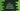# while loop in C++ Explanation with examples## Introduction :

while loop is similar to for loops. Using a loop, you can execute a piece of code repeatedly until a condition fails. In this blog post, we will learn how to use while loop in C++ with examples.

### Definition :

while keyword is used for a while loop. It checks one condition first and if that condition is true, it runs one piece of code written inside curly braces. The definition of while loop is as below :

``````while(condition){
// code
}``````

On next iteration, it will check for the condition again. If it is true again, it will run the same piece of code. So, we need to write the program in such a way that the condition will be false at some point of time. Otherwise, it will keep running for indefinite amount of time.

### Example of while loop in C++ :

The following program uses one while loop that prints all even numbers from 1 to 10 :

``````#include <iostream>
using namespace std;

int main()
{
int current = 1;

while (current < 11)
{
if (current % 2 == 0)
{
cout << current << endl;
}
current++;
}
}``````

Here, the while loop will run until the value of current is equal or greater than 11. Inside the loop, we are incrementing the value current by 1 on each iteration. Also, if the value of current is even, we are printing this value. If you run this program, it will print the below output :

### Infinite while loop :

I have mentioned before that an infinite while loop runs for indefinite amount of time. Let me show you with an example how it looks like :

``````#include <iostream>
using namespace std;

int main()
{
while (true)
{
cout<<"running..."<<endl;
}
}``````

It will keep running continuously because our condition is true that will always execute the loop. You will have to forcefully close the app using ctrl + c.

In production applications it is not a good idea to use infinite while loop because it may produce out of memory error.